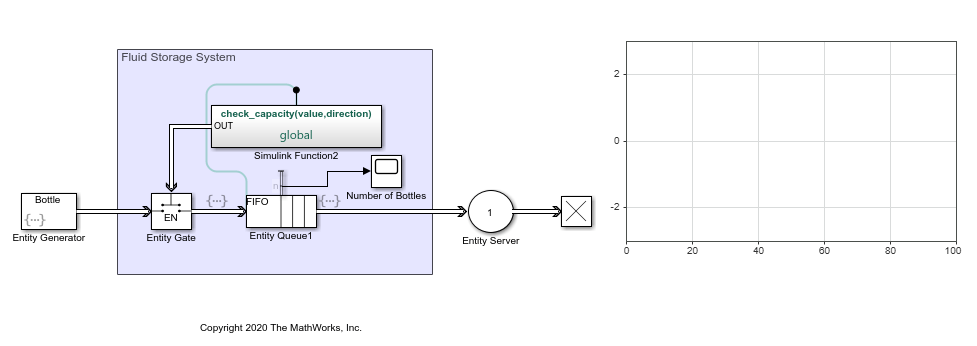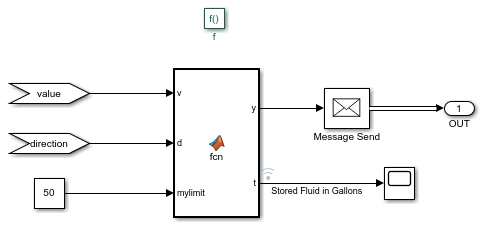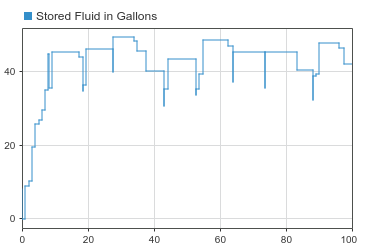# Use Queue Event Actions to Model a Storage Tank

This example shows how to use Entity Queue block event actions, a Simulink Function block, and an Entity Gate block to model a bottle storage system with a limited capacity.

This example uses Entity Generator, Entity Queue, Entity Server, and Entity Terminator blocks to model an assembly line for a bottle storage system with a limited weight capacity. The Entity Generator block represents the arrival of the bottles. Each bottle has a `gallons` attribute, whose value ranges from `0` and `10`, to indicate the amount of liquid it carries. The Entity Queue block represents the storage with limited capacity. The Entity Server block represents the processing of the bottles before they leave the facility.

A fluid storage control system is deployed by using a Simulink Function block and an Entity Gate block to allow bottles to enter the storage system as long as total stored gallon amount is less than `50` gallons. This amount corresponds to the maximum allowed weight the storage can support. It is assumed that the bottle weight is negligible. The Entity Gate block has two states: open and closed. The Simulink Function block monitors the amount of liquid in the storage and controls the state of the Entity Gate block.### Construct the Fluid Storage Control System using Queue Event Actions

The goal of constructing the Fluid Storage Control System is to allow bottles to enter the queue's storage as long as the total storage gallon amount does not exceed `50` gallons.

To construct the Fluid Storage Control System:

• A Simulink Function block is used with the function signature `check_capacity(value,direction)` to control the Entity Gate block. When the block sends a message with value `1`, the Enable Gate opens to allow containers into the storage. Otherwise, when the block sends a message with value `0`, the gate remains closed.

• In the Entity Queue block, in the Entry action and Exit action fields, `check_capacity(entity.gallons,1)` and `check_capacity(entity.gallons,-1)` send the container gallon value to the Simulink Function block. The second input of `check_capacity(~,~)` function takes value `1` when an entity enters the queue and `-1` when an entity exits the queue.

• In the Simulink Function block, a MATLAB Function block creates the logic to control the gate. The block accepts three inputs.

1. `v` is the value of the `gallon` attribute for each entity that enters or exits the block.

2. `direction` takes value `1` or `-1` to indicate if an entity enters or exits the block.

3. `myLimit` is the capacity of the liquid container in gallons.• The MATLAB Function block has two outputs.

1. `y` takes the value `1` to open the gate, when there is enough storage, and `0` otherwise.

2. `t` is the number of gallons of liquid in the storage.

• The MATLAB Function block contains the following logic to open and close the gate.

```function [y,t] = fcn(v,d,myLimit) % Initialize the persistent variable total that represents the total % number of gallons of liquid in the storage. persistent total % Initialize the variable total. if isempty(total) total = 0; end % Add or subtract the gallons carried by the entering or exiting % entity. d is 1 if the entitiy enters the storage and -1 otherwise. total = total + d*v; % Update total number of gallons in the storage. t = total; % Compare the total value with myLimit. Containers can have maximum 10 % gallons of liquid. if total > (myLimit-10) % Keep the gate closed. y = 0; else % Open the gate to allow containers. y = 1; end end ```

### Simulate the Model and Review Results

Simulate the model. Observe the number of containers in the liquid storage.Also, observe the amount of liquid in the storage throughout the simulation, which does not exceed `50` gallons.